Nutrition at Starbucks, Part I. (8.22, p. 326) The scatterplot below shows the relationship between the number of calories and amount of carbohydrates (in grams) Starbucks food menu items contain. Since Starbucks only lists the number of calories on the display items, we are interested in predicting the amount of carbs a menu item has based on its calorie content.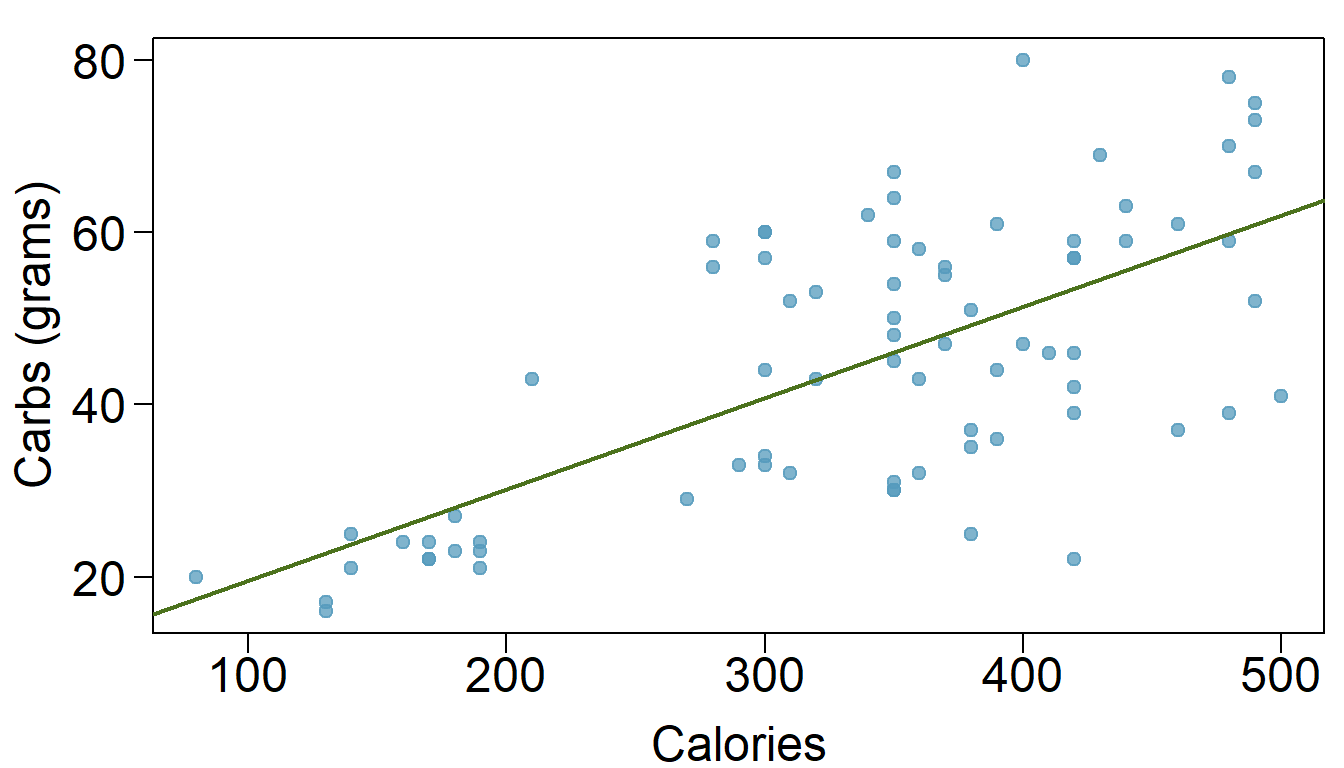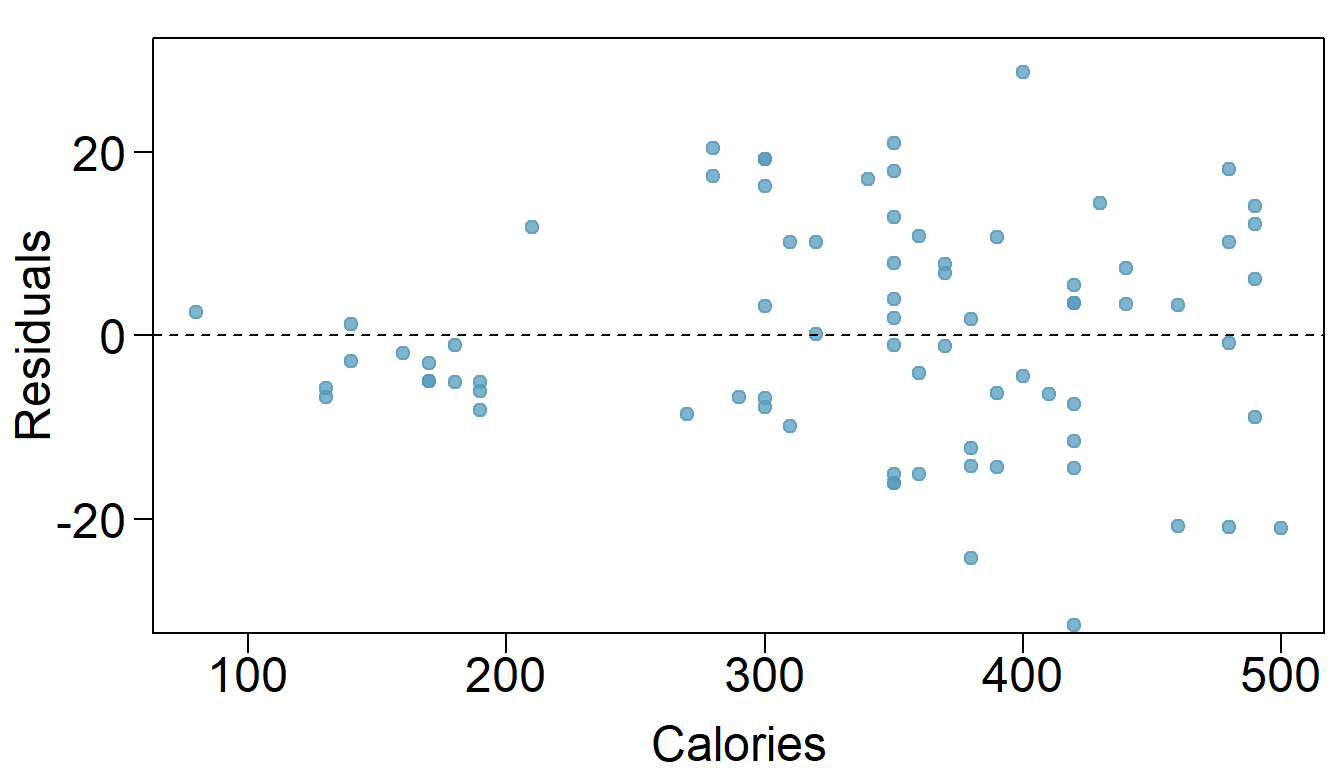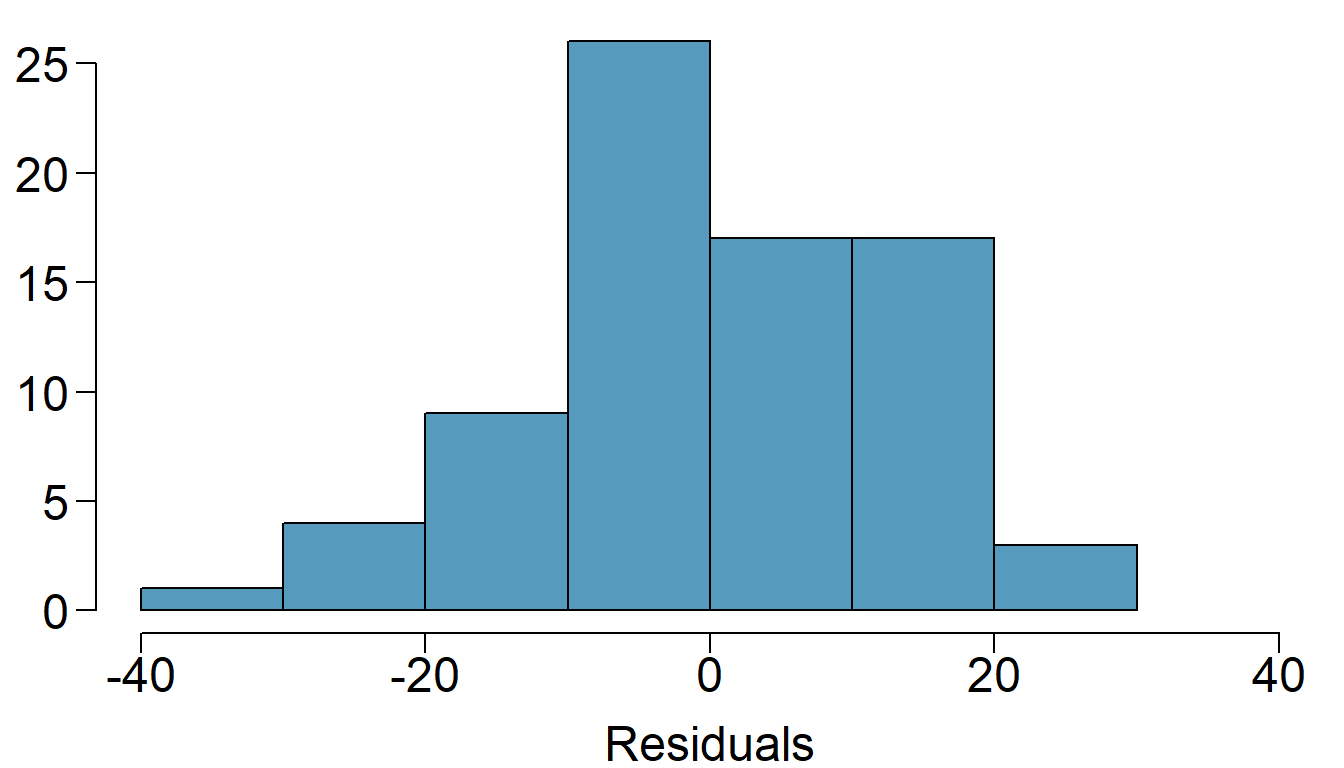1. Describe the relationship between number of calories and amount of carbohydrates (in grams) that Starbucks food menu items contain.

The number of calories and the amount of carbohydrates seems to have a positive relationship. As calories increase, carbohydrates also increase.

1. In this scenario, what are the explanatory and response variables?

Calories is the explanatory variable and Carbohydrates is the Response variable.

1. Why might we want to fit a regression line to these data?

We can determine the amount of carbs a menu item has based on its calorie content.

1. Do these data meet the conditions required for fitting a least squares line?

The data is somewhat tricky; it seems to meet the conditions for fitting a least squares line (linearity, Nearly normal residuals, constant variability and independent observations), but there is some variability as calories increase.

Body measurements, Part I. (8.13, p. 316) Researchers studying anthropometry collected body girth measurements and skeletal diameter measurements, as well as age, weight, height and gender for 507 physically active individuals.19 The scatterplot below shows the relationship between height and shoulder girth (over deltoid muscles), both measured in centimeters.

\begin{center}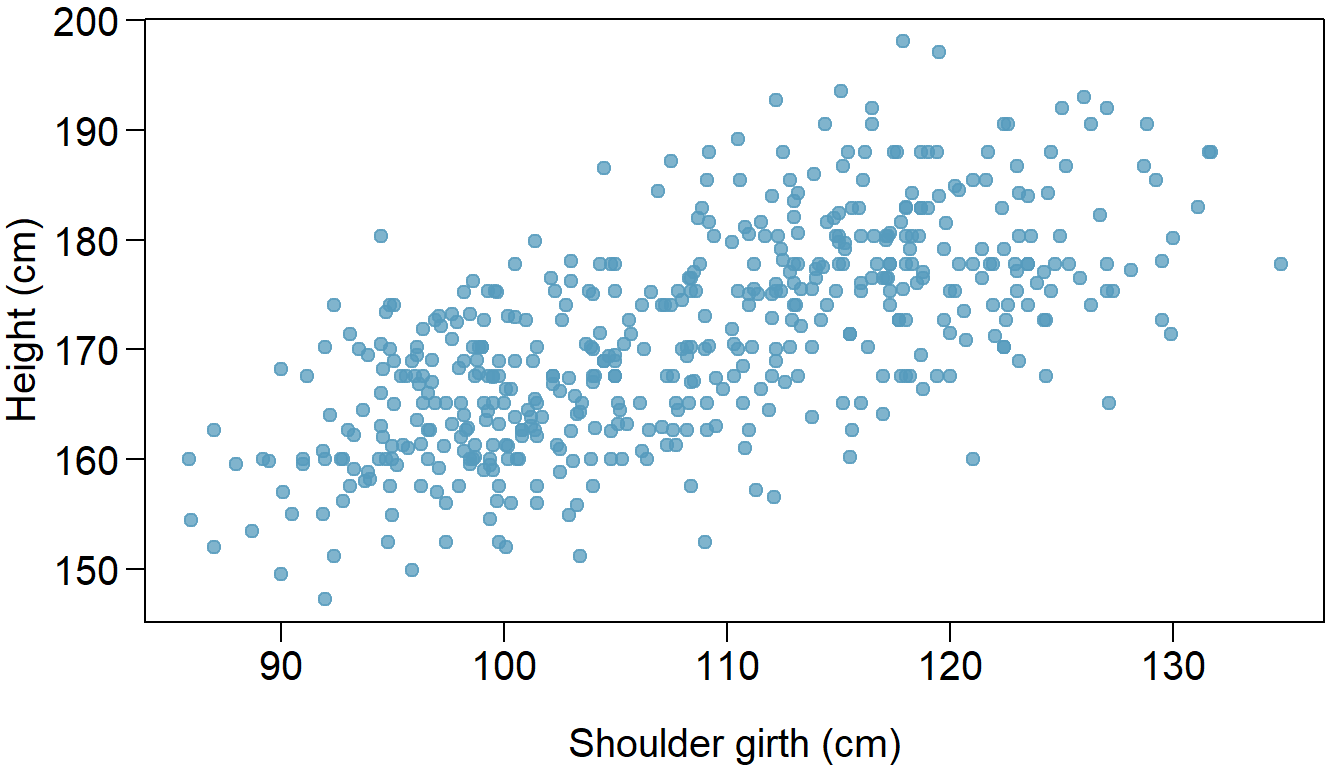\end{center}

1. Describe the relationship between shoulder girth and height.

It looks like there is a positive linear relationship between shoulder girth and height.

1. How would the relationship change if shoulder girth was measured in inches while the units of height remained in centimeters?

In this case, the shoulder girth dimension will be shifted to the right, which will shift all the points to the right, but the linearity will still be there, and therefore the relationship will be the same.

Body measurements, Part III. (8.24, p. 326) Exercise above introduces data on shoulder girth and height of a group of individuals. The mean shoulder girth is 107.20 cm with a standard deviation of 10.37 cm. The mean height is 171.14 cm with a standard deviation of 9.41 cm. The correlation between height and shoulder girth is 0.67.

1. Write the equation of the regression line for predicting height.
sy <- 9.41
sx <- 10.37
xbar <- 107.2
ybar <- 171.14
R <- 0.67

b1 <- sy/sx * R
b0 <- b1 * -xbar + ybar

b1
##  0.6079749
b0
##  105.9651

The equation for the regression line for predicting height is:

y = 105.9651 + 0.6079749 * x

1. Interpret the slope and the intercept in this context.

Slope: Represents the number of centimeters increase in height for each increase in shoulder girth.

Intercept: Represent the height in centimeters at girth of 0 cm.

1. Calculate $$R^2$$ of the regression line for predicting height from shoulder girth, and interpret it in the context of the application.
R^2
##  0.4489

About 45% of the variability in height is account for by shoulder girth.

1. A randomly selected student from your class has a shoulder girth of 100 cm. Predict the height of this student using the model.
x <- 100

y <- b0 + b1 * x

y
##  166.7626

The height predicted for this student is: 166.76 cm.

1. The student from part (d) is 160 cm tall. Calculate the residual, and explain what this residual means.
i = 100 # from  part d
yi <- 160

ei <- yi - y

ei
##  -6.762581

The residual for this observation is: -6.762581.

This means that the actual data point is below the linear regression line and that the model is overestimating the value.

1. A one year old has a shoulder girth of 56 cm. Would it be appropriate to use this linear model to predict the height of this child?

It would not be appropriate to predict the hight of this child, because 56 cm is outside of the range of the shoulder grith which is between 85 cm and 135 cm.

Cats, Part I. (8.26, p. 327) The following regression output is for predicting the heart weight (in g) of cats from their body weight (in kg). The coefficients are estimated using a dataset of 144 domestic cats.

\begin{center}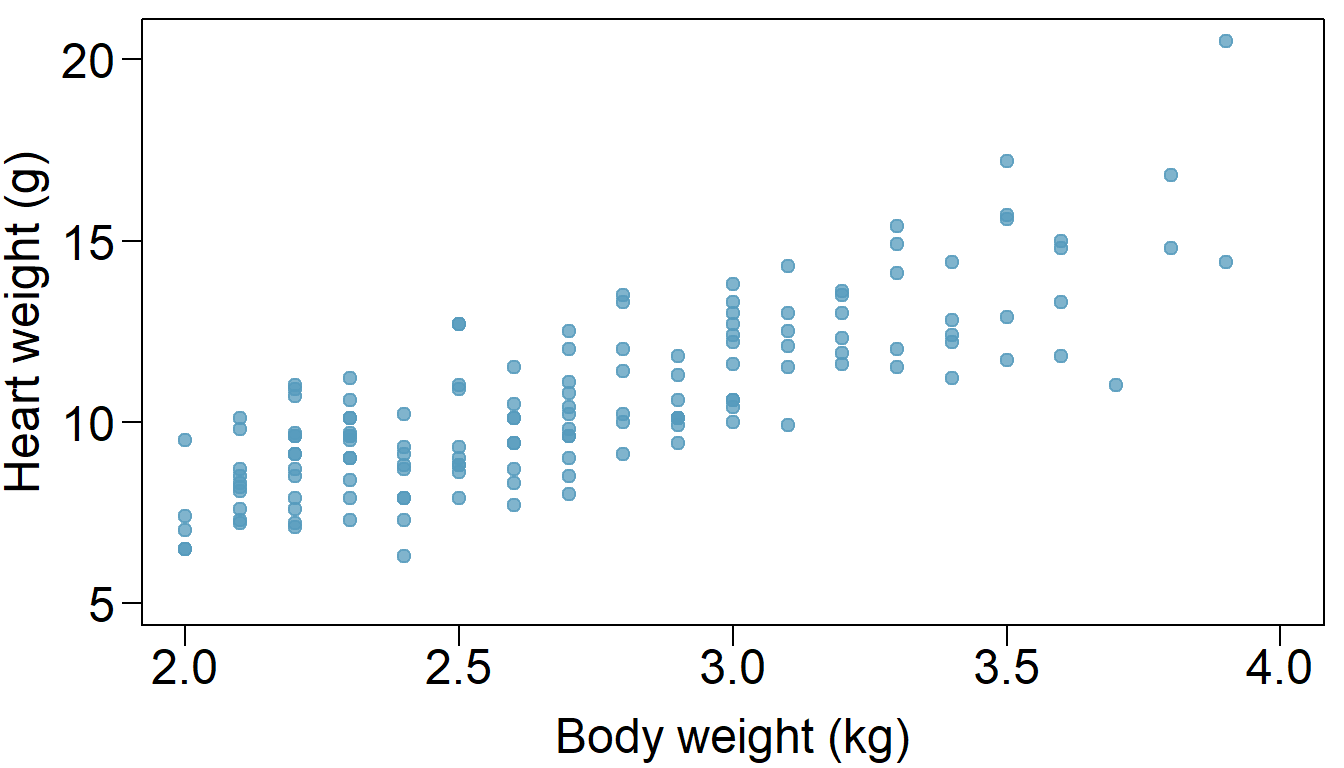\end{center}

1. Write out the linear model.

y = −0.357 + 4.034 * x

1. Interpret the intercept.

The intercept will be at -0.357. This value tells us that this model will predict a negative heart weight when the cat’s body weight is 0. In reality, this does not make since when x = 0.

1. Interpret the slope.

the slope of 4.034 tells us that the heart weight increases by 4.034 grams for each 1kg of body weight increase.

1. Interpret $$R^2$$.

the $$R^2$$ = 64.66% tells us that the linear model describes 64.66% of the variation in the heart weight.

1. Calculate the correlation coefficient.
R2 <- 0.6466

R <- sqrt(R2)

R
##  0.8041144

Our correlation coefficient is therefore 0.8041144

Rate my professor. (8.44, p. 340) Many college courses conclude by giving students the opportunity to evaluate the course and the instructor anonymously. However, the use of these student evaluations as an indicator of course quality and teaching effectiveness is often criticized because these measures may reflect the influence of non-teaching related characteristics, such as the physical appearance of the instructor. Researchers at University of Texas, Austin collected data on teaching evaluation score (higher score means better) and standardized beauty score (a score of 0 means average, negative score means below average, and a positive score means above average) for a sample of 463 professors. The scatterplot below shows the relationship between these variables, and also provided is a regression output for predicting teaching evaluation score from beauty score.

\begin{center}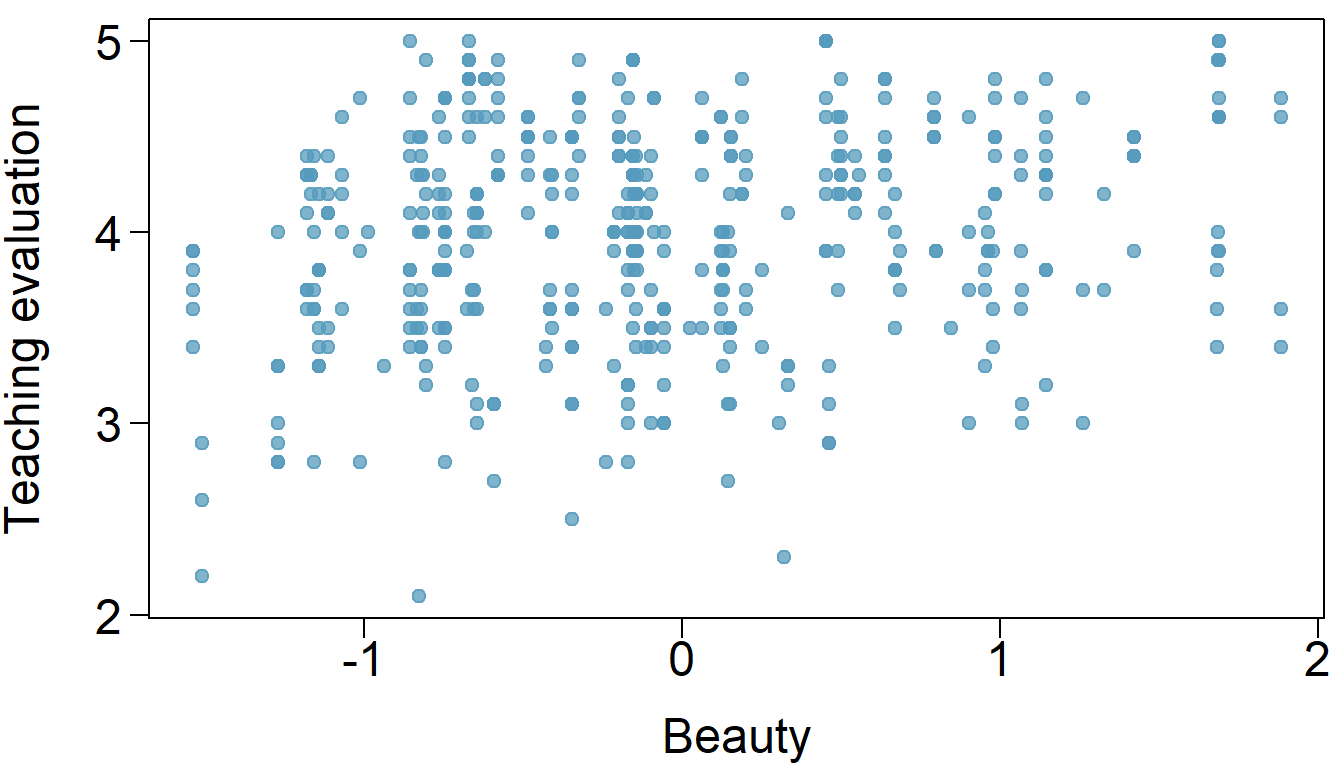\end{center}

1. Given that the average standardized beauty score is -0.0883 and average teaching evaluation score is 3.9983, calculate the slope. Alternatively, the slope may be computed using just the information provided in the model summary table.

We have y = b0 + b1 * x

b0 <- 4.010

x <- -0.0883
y <- 3.9983

b1 <- (y - b0) / x

b1
##  0.1325028

The slope is 0.1325028.

1. Do these data provide convincing evidence that the slope of the relationship between teaching evaluation and beauty is positive? Explain your reasoning.

We have from above: b1 > 0 => b1 = (Sy/Sx).R > 0. Since Sy > 0 and Sx > 0, we can conclude that the data provide convincing evidence that the slope of the relationship between teaching evaluation and beauty is positive.

1. List the conditions required for linear regression and check if each one is satisfied for this model based on the following diagnostic plots.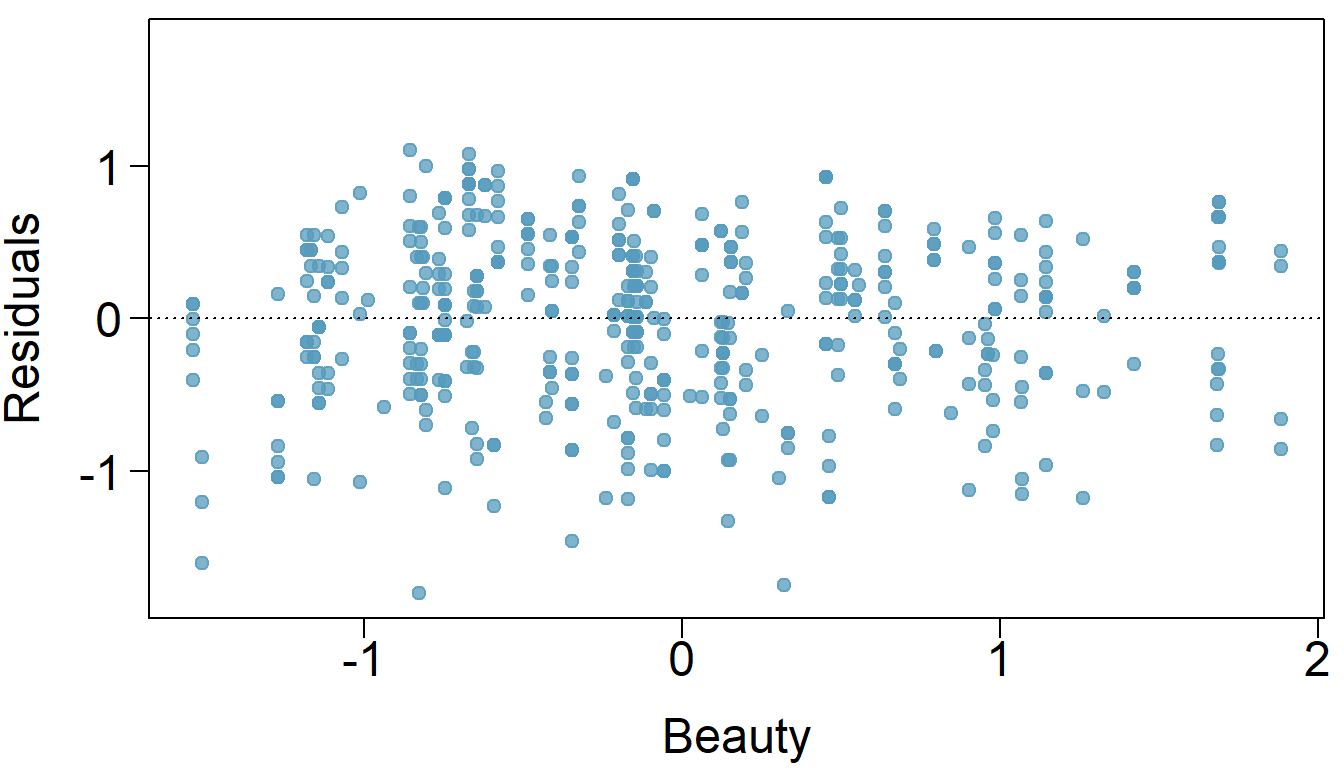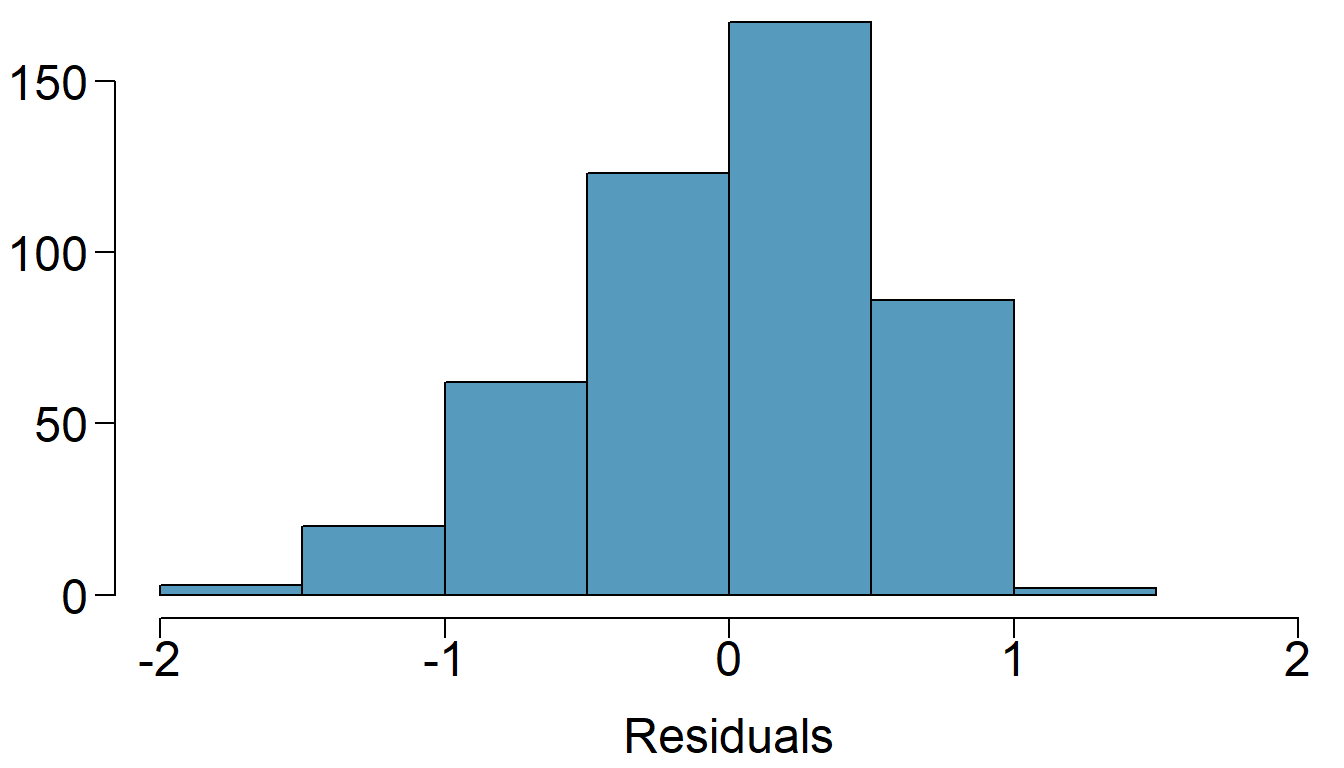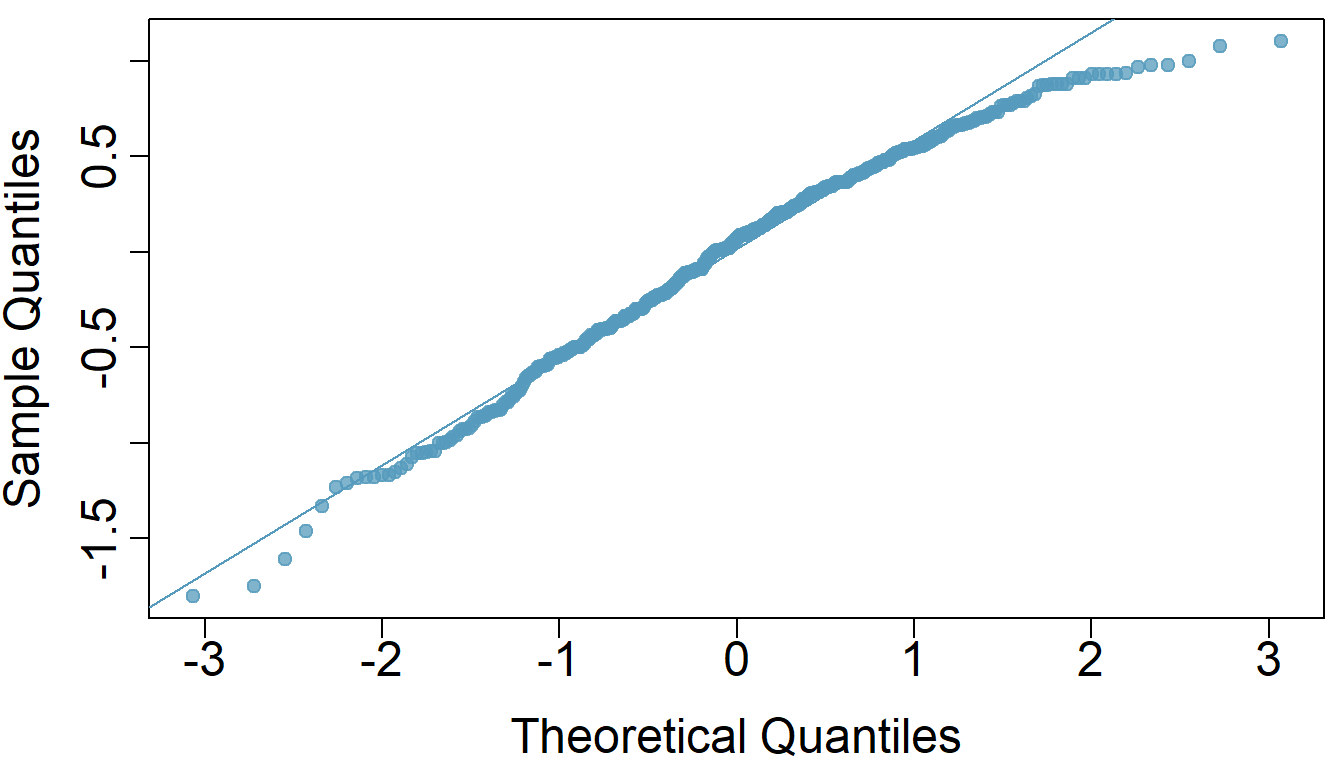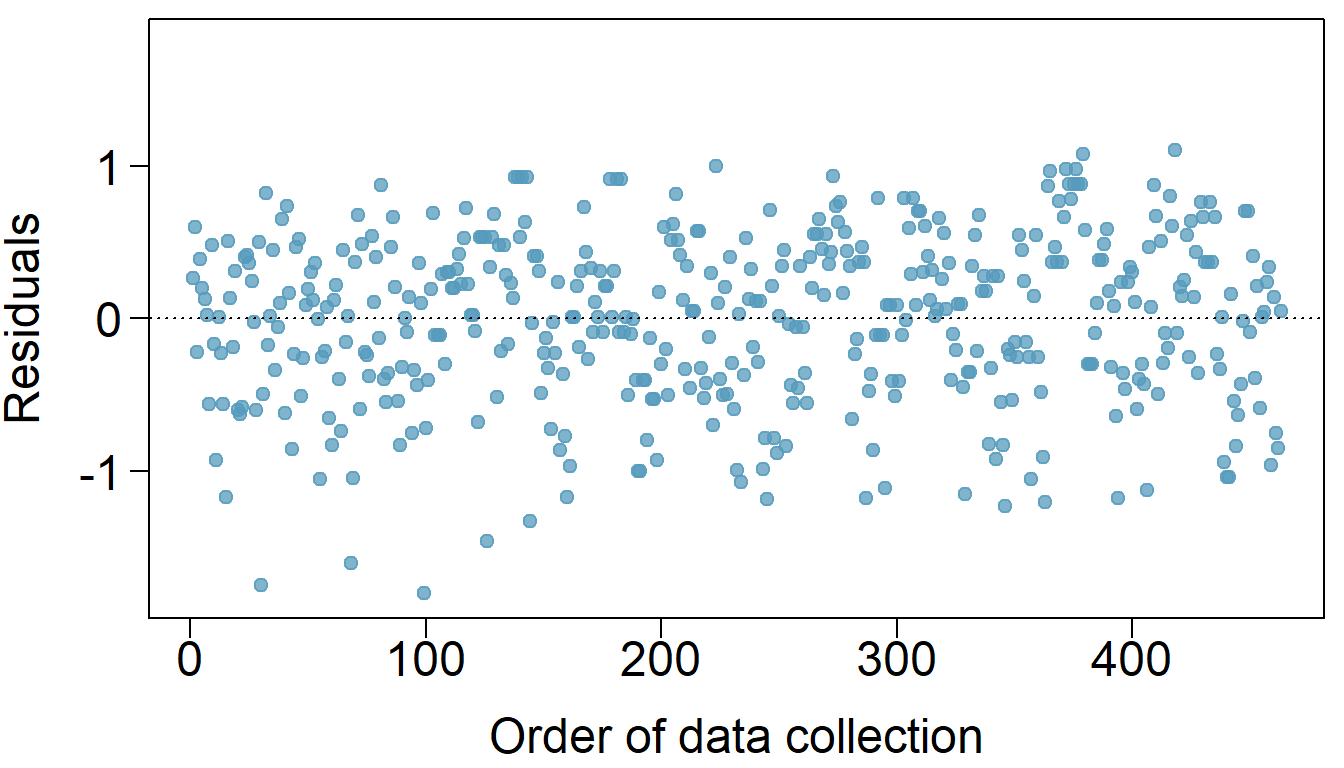Linearity: It’s not certain that we are seeing a linear trend.

Nearly normal residuals: The residuals is not normal and is skewed.

Constant variability: The scatterplot of the residuals does appear to have constant variability.

Independent observations: Assuming independence due to no clear evidence one way or the other. 463 professors is in fact < 10% of nationwide professors